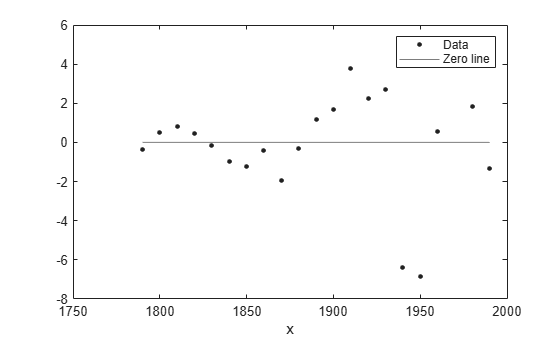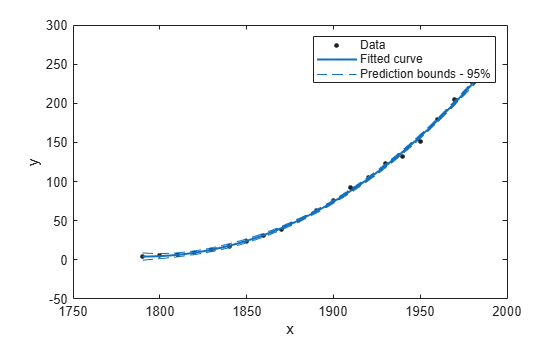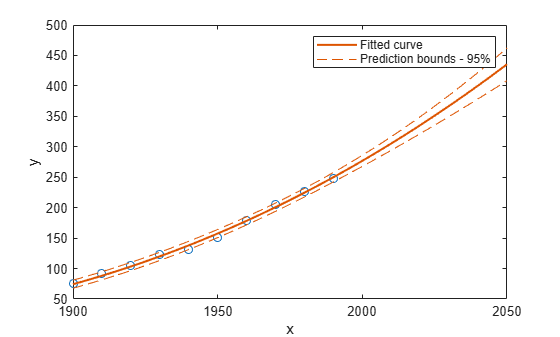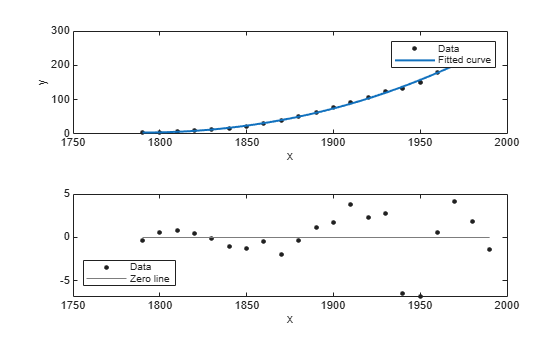Documentation

### This is machine translation

Mouseover text to see original. Click the button below to return to the English version of the page.

## Evaluate a Curve Fit

This example shows how to work with a curve fit.

### Load Data and Fit a Polynomial Curve

```load census curvefit = fit(cdate,pop,'poly3','normalize','on')```
```curvefit = Linear model Poly3: curvefit(x) = p1*x^3 + p2*x^2 + p3*x + p4 where x is normalized by mean 1890 and std 62.05 Coefficients (with 95% confidence bounds): p1 = 0.921 (-0.9743, 2.816) p2 = 25.18 (23.57, 26.79) p3 = 73.86 (70.33, 77.39) p4 = 61.74 (59.69, 63.8) ```

The output displays the fitted model equation, the fitted coefficients, and the confidence bounds for the fitted coefficients.

### Plot the Fit, Data, Residuals, and Prediction Bounds

`plot(curvefit,cdate,pop)`Plot the residuals fit.

`plot(curvefit,cdate,pop,'Residuals')`Plot the prediction bounds on the fit.

`plot(curvefit,cdate,pop,'predfunc')`### Evaluate the Fit at a Specified Point

Evaluate the fit at a specific point by specifying a value for `x` , using this form: `y = fittedmodel(x)`.

`curvefit(1991)`
```ans = 252.6690 ```

### Evaluate the Fit Values at Many Points

Evaluate the model at a vector of values to extrapolate to the year 2050.

```xi = (2000:10:2050).'; curvefit(xi)```
```ans = 6×1 276.9632 305.4420 335.5066 367.1802 400.4859 435.4468 ```

Get prediction bounds on those values.

`ci = predint(curvefit,xi)`
```ci = 6×2 267.8589 286.0674 294.3070 316.5770 321.5924 349.4208 349.7275 384.6329 378.7255 422.2462 408.5919 462.3017 ```

Plot the fit and prediction intervals across the extrapolated fit range. By default, the fit is plotted over the range of the data. To see values extrapolated from the fit, set the upper x-limit of the axes to 2050 before plotting the fit. To plot prediction intervals, use `predobs` or `predfun` as the plot type.

```plot(cdate,pop,'o') xlim([1900,2050]) hold on plot(curvefit,'predobs') hold off```### Get the Model Equation

Enter the fit name to display the model equation, the fitted coefficients, and the confidence bounds for the fitted coefficients.

`curvefit`
```curvefit = Linear model Poly3: curvefit(x) = p1*x^3 + p2*x^2 + p3*x + p4 where x is normalized by mean 1890 and std 62.05 Coefficients (with 95% confidence bounds): p1 = 0.921 (-0.9743, 2.816) p2 = 25.18 (23.57, 26.79) p3 = 73.86 (70.33, 77.39) p4 = 61.74 (59.69, 63.8) ```

To get only the model equation, use `formula`.

`formula(curvefit)`
```ans = 'p1*x^3 + p2*x^2 + p3*x + p4' ```

### Get Coefficient Names and Values

Specify a coefficient by name.

`p1 = curvefit.p1`
```p1 = 0.9210 ```
`p2 = curvefit.p2`
```p2 = 25.1834 ```

Get all the coefficient names. Look at the fit equation (for example, `f(x) = p1*x^3+...` ) to see the model terms for each coefficient.

`coeffnames(curvefit)`
```ans = 4x1 cell array {'p1'} {'p2'} {'p3'} {'p4'} ```

Get all the coefficient values.

`coeffvalues(curvefit)`
```ans = 1×4 0.9210 25.1834 73.8598 61.7444 ```

### Get Confidence Bounds on the Coefficients

Use confidence bounds on coefficients to help you evaluate and compare fits. The confidence bounds on the coefficients determine their accuracy. Bounds that are far apart indicate uncertainty. If the bounds cross zero for linear coefficients, this means you cannot be sure that these coefficients differ from zero. If some model terms have coefficients of zero, then they are not helping with the fit.

`confint(curvefit)`
```ans = 2×4 -0.9743 23.5736 70.3308 59.6907 2.8163 26.7931 77.3888 63.7981 ```

### Examine Goodness-of-Fit Statistics

To get goodness-of-fit statistics at the command line, you can either:

• Open Curve Fitting app and select Fit > Save to Workspace to export your fit and goodness of fit to the workspace.

• Specify the `gof` output argument using the `fit` function.

Recreate the fit specifying the `gof` and output arguments to get goodness-of-fit statistics and fitting algorithm information.

`[curvefit,gof,output] = fit(cdate,pop,'poly3','normalize','on')`
```curvefit = Linear model Poly3: curvefit(x) = p1*x^3 + p2*x^2 + p3*x + p4 where x is normalized by mean 1890 and std 62.05 Coefficients (with 95% confidence bounds): p1 = 0.921 (-0.9743, 2.816) p2 = 25.18 (23.57, 26.79) p3 = 73.86 (70.33, 77.39) p4 = 61.74 (59.69, 63.8) ```
```gof = struct with fields: sse: 149.7687 rsquare: 0.9988 dfe: 17 adjrsquare: 0.9986 rmse: 2.9682 ```
```output = struct with fields: numobs: 21 numparam: 4 residuals: [21x1 double] Jacobian: [21x4 double] exitflag: 1 algorithm: 'QR factorization and solve' iterations: 1 ```

Plot a histogram of the residuals to look for a roughly normal distribution.

`histogram(output.residuals,10)`### Plot the Fit, Data, and Residuals

```plot(curvefit,cdate,pop,'fit','residuals') legend Location SouthWest subplot(2,1,1) legend Location NorthWest```### Find Methods

List every method that you can use with the fit.

`methods(curvefit)`
```Methods for class cfit: argnames confint formula numcoeffs setoptions category dependnames indepnames plot type cfit differentiate integrate predint coeffnames feval islinear probnames coeffvalues fitoptions numargs probvalues ```

Use the `help` command to find out how to use a fit method.

`help cfit/differentiate`
``` DIFFERENTIATE Differentiate a fit result object. DERIV1 = DIFFERENTIATE(FITOBJ,X) differentiates the model FITOBJ at the points specified by X and returns the result in DERIV1. FITOBJ is a Fit object generated by the FIT or CFIT function. X is a vector. DERIV1 is a vector with the same size as X. Mathematically speaking, DERIV1 = D(FITOBJ)/D(X). [DERIV1,DERIV2] = DIFFERENTIATE(FITOBJ, X) computes the first and second derivatives, DERIV1 and DERIV2 respectively, of the model FITOBJ. See also CFIT/INTEGRATE, FIT, CFIT. ```